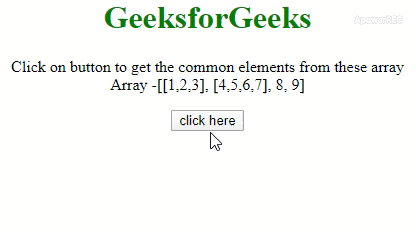Open in App
Not now

# How to flatten array with the JavaScript/jQuery ?

• Last Updated : 02 Mar, 2020

JavaScript contains many arrays (or 2-d array) and the task is to flatten the array and make that look like 1-d JavaScript array. There are two approaches that are discussed below. You can also use Underscore.js _.flatten() with Examples.

Approach 1: Use Array.prototype.concat.apply() method to perform the operation. The concat() and apply() method is used to concat the arrays to 1-d array.

• Example:

 ` ` `<``html``> ` ` `  `<``head``> ` `    ``<``title``> ` `        ``How to flatten array with ` `        ``the JavaScript? ` `    `` ` `     `  `    ``<``style``> ` `        ``body { ` `            ``text-align: center; ` `        ``} ` `         `  `        ``h1 { ` `            ``color: green; ` `        ``} ` `    `` ` ` ` ` `  `<``body``> ` `    ``<``h1``>GeeksforGeeks ` `     `  `    ``<``p` `id``=``"GFG_UP"``> ` `     `  `    ``<``button` `onClick``=``"GFG_Fun()"``> ` `        ``click here ` `    `` ` `     `  `    ``<``p` `id``=``"GFG_DOWN"``> ` `     `  `    ``<``script``> ` `        ``var up = document.getElementById('GFG_UP'); ` `        ``var down = document.getElementById('GFG_DOWN'); ` `        ``var arr1 = [1, 2, 3]; ` `        ``var arr2 = [4, 5, 6, 7]; ` `        ``var arr = [arr1, arr2, 8, 9]; ` `         `  `        ``up.innerHTML = "Click on button to get " ` `                ``+ "the common elements from these" ` `                ``+ " array <``br``>Array -[[" + arr ` `                ``+ "], [" + arr + "], " + arr ` `                ``+ ", " + arr + "]"; ` ` `  `        ``function GFG_Fun() { ` `            ``down.innerHTML =  ` `                ``Array.prototype.concat.apply([], arr); ` `        ``} ` `    `` ` ` ` ` `  ` `

• Output:Approach 2: The \$.map() method in jQuery can be used to perform the operation. This method takes the array and a method as input. The second argument which is a method takes elements of original array one by one and return its elements.

• Example:

 ` ` `<``html``> ` ` `  `<``head``> ` `    ``<``title``> ` `        ``How to flatten array with ` `        ``the JavaScript? ` `    `` ` `     `  `    ``<``style``> ` `        ``body { ` `            ``text-align: center; ` `        ``} ` `         `  `        ``h1 { ` `            ``color: green; ` `        ``} ` `    `` ` `    ``<``script` `src``= ` `"https://ajax.googleapis.com/ajax/libs/jquery/3.4.0/jquery.min.js"``> ` `    `` ` ` ` ` `  `<``body``> ` `    ``<``h1``>GeeksforGeeks ` `     `  `    ``<``p` `id``=``"GFG_UP"``> ` `     `  `    ``<``button` `onClick``=``"GFG_Fun()"``> ` `        ``click here ` `    `` ` `     `  `    ``<``p` `id``=``"GFG_DOWN"``> ` `     `  `    ``<``script``> ` `        ``var up = document.getElementById('GFG_UP'); ` `        ``var down = document.getElementById('GFG_DOWN'); ` `        ``var arr1 = [1, 2, 3]; ` `        ``var arr2 = [4, 5, 6, 7]; ` `        ``var arr = [arr1, arr2, 8, 9]; ` `         `  `        ``up.innerHTML = "Click on button to get the" ` `                ``+ " common elements from these array" ` `                ``+ "<``br``>Array - [[" + arr + "], [" ` `                ``+ arr + "], " + arr + ", "  ` `                ``+ arr + "]"; ` ` `  `        ``function GFG_Fun() { ` `            ``down.innerHTML = \$.map(arr, function(n) { ` `                ``return n; ` `            ``}); ` `        ``} ` `    `` ` ` ` ` `  ` `

• Output:My Personal Notes arrow_drop_up
Related Articles